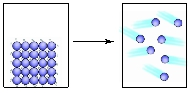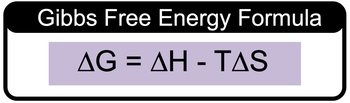## GOB Chemistry

Learn the toughest concepts covered in your GOB - General, Organic, and Biological Chemistry class with step-by-step video tutorials and practice problems.

Energy, Rate and Equilibrium

# Gibbs Free Energy (Simplified)

Gibbs Free Energy represents energy associated with a chemical reaction that can be used to do work.

Gibbs Free Energy
1
concept

## Gibbs Free Energy (Simplified) Concept 146s
Play a video:
Gibbs free energy, which is delta G is a measure of energy change of a chemical or physical process that can be used to do work. Now we're going to say, the sign of delta G determines the spontaneity of a reaction. All right, so we're gonna say when our delta G value is less than zero, meaning that it's negative. That means our reaction is spontaneous when it's the opposite. When delta G is greater than zero, so it's positive, it becomes non spontaneous, and then finally, when delta G is equal to zero, that means we are at equilibrium. So we're neither spontaneous or non spontaneous or right on the border. So, just remember the sign of delta G can determine the spontaneity of a chemical reaction.
2
example

## Gibbs Free Energy (Simplified) Example 11m
Play a video:
if delta G is small and positive, which of the following statements is true. So before we solve this question, realized that we're dealing with reversible reactions so they can go in the four directions or the reverse direction. Now they're telling us delta G is small, so that means it's close to zero and it's positive. So that means it's slightly greater than zero, which means it's non spontaneous. And when we say that we mean non spontaneous in the forward direction, but remember we're dealing with reversible reactions when we talk about gibbs free energy. So if you're non spontaneous in the four direction, that means that you are spontaneous in the reverse. So let's see what makes the most sense. So it's positive. So that means it's going to be non spontaneous in the forward. Which means that A and B are out because it wouldn't be spontaneous in the forward. It will be spontaneous though in the reverse, which is what was said. But now, is it far from equilibrium or near equilibrium? Remember delta G. When it's equal to zero, we are at equilibrium, since delta G is small, that means it's a number that's pretty close to zero, so that means we would be near equilibrium. This would mean that option D is the correct answer.
3
concept

## Gibbs Free Energy (Simplified) Concept 21m
Play a video:
when the sign of delta G. Is unknown, we can say that the spontaneity of a reaction came predicted from the sides of entropy which is delta H. And entropy which is DELTA. S. So here we're going to say that when they're both positive we are spontaneous at high temperatures. And if both of them being positive makes it spontaneous at high temperatures, then what happens when it's the opposite? Well, when they're both negative, we'd be spontaneous at low temperatures. Natural, willing to say when DELTA H. Is positive and delta S. Is negative. You are always non spontaneous in terms of your chemical reaction. And if we do the opposite of then if DELTA F. H is now negative and delta as is positive, then you'd be spontaneous for your chemical reaction. So just remember the easiest way to remember this here. You're adults at H positive and negative, huge adult S. Positive and negative. So you'd say hi t low t non and spawn. So the sides of our delta and DELTA S. For chemical reaction can be used to determine the right temperature conditions to make it spontaneous or not.
4
example

## Gibbs Free Energy (Simplified) Example 22m
Play a video:
here we're going to say that PCL three phosphorus try chloride plus chorine gas react together to give us phosphorus Penta chloride At 25°C. The entropy is negative 92.50 killer jewels. Which are the following statements is our true. This is an indo thermic reaction. Well, DELTA H here is negative. So it is excel thermic not in no thermic, if the temperatures increase, the ratio of our products, Overreactions will increase. Alright, so first of all we're going to say products Overreactions is talking about our equilibrium expression. So we're talking about our equilibrium constant K. Next we're going to say is let's determine the sign of DELTA H. And DELTA S. So delta H positive negative, delta S, positive and negative. We know that delta H. Is negative because we're told that right from the beginning, so we're dealing with this one and then what you're gonna say, you can determine the sign of delta S. We have to react ints combined to give us one product. So we're forming bonds, which means that delta assets negative. So that means we'd fit in this slot, which means that are as temperatures decrease, we become more spontaneous. Okay, So we're spontaneous and low temperatures. So if we're increasing the temperature, we're increasing the temperature, that's going to have the opposite effect, it's gonna make us less spontaneous and more non spontaneous and realize what we're making a reaction more non spontaneous. That means it doesn't want to happen. That means the reverse direction is favored. So that means that reactant are favored more than products. Okay, so remember K equals products. Overreact ints. Yeah, we're heading in the reverse direction where reactions become more favored. So your bottom part is becoming bigger and as a consequence of top part is becoming smaller. So overall what's happening to K. K would be decreasing. So this is not true here. The Delta asked for the reaction is negative. Yes. What multiple reactions combined to give me one product that is a decrease in entropy, so it's negative. And DELTA G for the reaction has to be negative at all temperatures. No, it's only going to be negative or spontaneous at low temperatures. So the only statement here that's true would be options. See.
5
Problem

What are the signs of ∆H, ∆S and ∆G for the spontaneous conversion of a solid into gas?6
Problem

Consider the combustion of butane gas and predict the signs of ΔS, ΔH and ∆G.

C4H10(g) + 13/2 O2(g) ⟶ 4 CO2(g) + 5 H2O(g)

7
concept

## Gibbs Free Energy (Simplified) Concept 31m
Play a video:
So we can calculate the value for gibbs free energy delta G. We're gonna say we do this by using gibbs free energy formula. It allows us to calculate the value of delta gene by using delta H. Usually it's given to us in kila jewels delta S. Which is usually given to us in joules per kelvin and temperature which has given us given to us in Calvin together. It gives us the formula delta G equals delta H minus T. Delta S. Now remember it's always important to include your units within your calculations and realize that if they give us delta H. And kill a jewels and delta S. And jewels, those units do not match. So you have to convert them so that they do match. So either make both units killed jules, it's or you make both units jewels. What's customary really done is that we convert everything to kill a jewels because that's more of a standard value to use for these types of calculations. So keep that in mind. And remember we can find delta G. By using this formula delta G equals delta H minus T. Delta S.8
example

## Gibbs Free Energy (Simplified) Example 31m
Play a video:
for particular reaction, Delta H equals negative 1 11.4 killer jewels. In delta S. Equals negative 25 joules per kelvin calculate delta G. For this reaction at 2200 and 98 kelvin. What can be said about the spontaneity of the reaction? At 298 kelvin. Alright, so delta jean equals delta H minus T. Delta S. Delta H is negative 1 11.4 killer jewels minus the temperature in kelvin, which is 2 98 kelvin. And then we have to convert our jewels to kill the jewels. Remember that just involves moving the decimal point over three. So that would be negative .025. Kill the jewels over kelvin, kelvin's cancel out. Your answer at the end will be in killer jewels When you plug that in, you're gonna get negative 103.95 killer jewels. Since your delta G value is less than zero, we can say that this reaction is spontaneous. So here we say, the the system is spontaneous as written, so the answer would be C. Not at equilibrium because delta you would have to equal zero and it would be spontaneous in the reverse direction. If we had gotten adult Iggy, That is greater than zero because we'd be non spontaneous in the four direction, and we have to go in the reverse direction to be spontaneous.
9
Problem

A particular reaction has ΔG = –350 kJ and ΔS = –350 J/K at 24°C. How much heat will be released/absorbed?

10
Problem

For a reaction in which ΔH = 125 kJ and ΔS = 325 J/K, determine the temperature in Celsius above which the reaction is spontaneous.